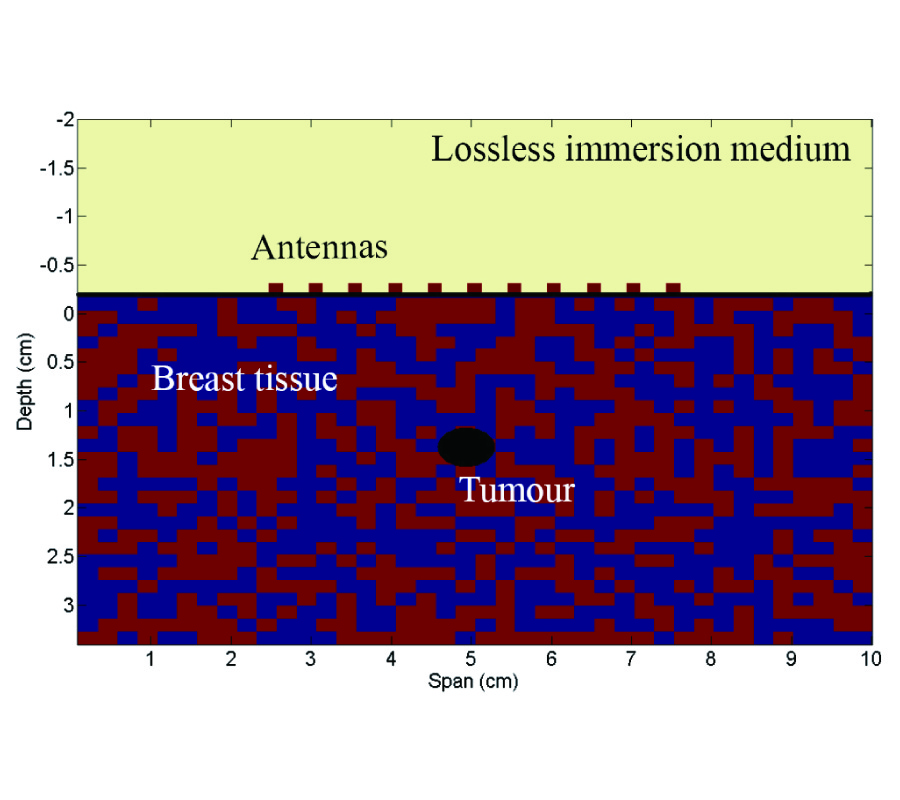Vol. 18
Latest Volume
All Volumes
All Journals
2009-11-28
PIER B
Vol. 18, 381-399, 2009
Ased-AIM Analysis of Scattering by Large-Scale Finite Periodic Arrays
Li Hu , Joshua Le-Wei Li and Tat Yeo
In this paper, the Adaptive Integral Method (AIM) has been extended to characterizing electromagnetic scattering by large scale finite periodic arrays with each cell comprising of either dielectric or metallic objects, by utilizing accurate sub-entire-domain (ASED) basis function. The solution process can be carried out in two steps. In the first step, a small problem is solved in order to construct ASED basis functions to be implemented for the second step. When dielectric materials are involved in the cell which results in a large number of unknowns for the small problem, the AIM can be used to accelerate the solution process and reduce the memory requirement. In the second step, the entire problem is solved using the ASED basis function constructed in the first step. The AIM can be enhanced with the ASED basis function implemented to solve the entire problem more efficiently. When calculating the near interaction impedance matrix, computation time can be significantly reduced by using the near impedance matrix in the first step. The complexity analysis shows that the computational time is O(N0 logN0) + O(M logM) and memory requirement is O(N0) + O(M), where N0 denotes the number of cells and M stands for the number of elements in one cell. The results calculated respectively by the ASED-AIM and the existing AIM are then compared and an excellent agreement has been observed, which demonstrates the accuracy of the proposed method. In the meantime, memory and computational time requirements have been considerably reduced using the ASED-AIM as compared to the existing AIM. Finally, an example with over 10 million unknowns is given to demonstrate the efficiency of the proposed method.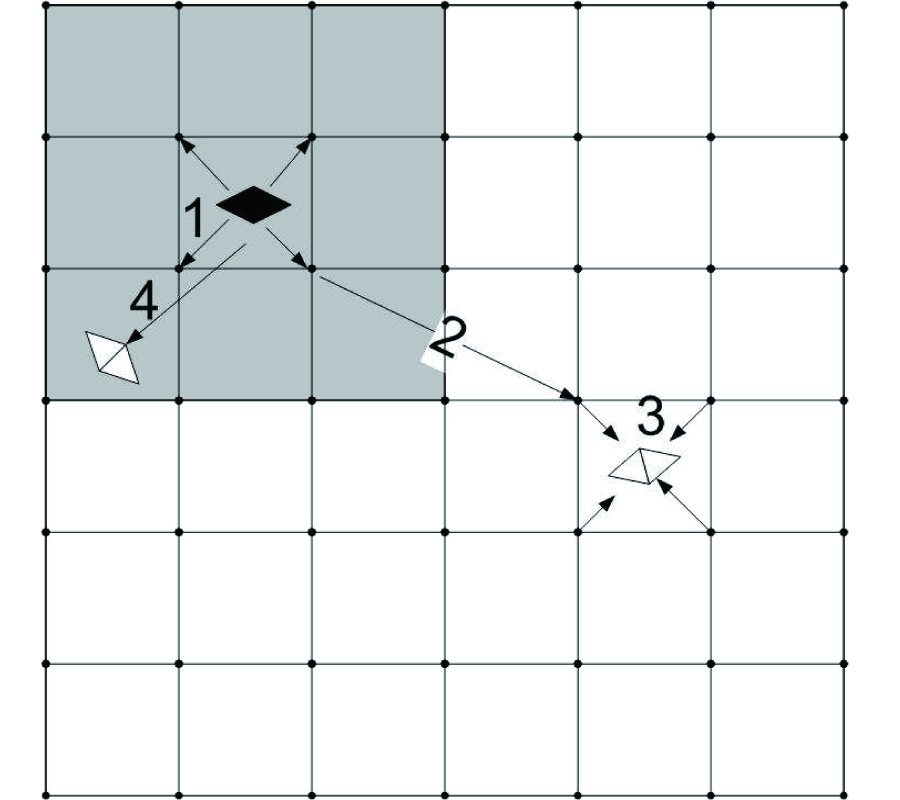2009-11-27
PIER B
Vol. 18, 365-380, 2009
Prediction of Probability Distribution of Electromagnetic Wave in Vdt Environment Based on Fuzzy Measurement Data Under Existence of Background Noise
Hisako Orimoto and Akira Ikuta
In this paper, based on fuzzy measurement data, a prediction method for probability distribution of electromagnetic wave leaked from electronic information equipment is proposed. More specifically, by applying the well-known probability measure of fuzzy events to the probability distribution in an orthogonal expansion series form reflecting systematically various types of correlation information, a method to estimate precisely the correlation information between the electromagnetic and sound waves from the conditional moment statistics of fuzzy variables is proposed under actual situation in the existence of a background noise. The effectiveness of the proposed theory is experimentally confirmed by applying it to the observation data leaked from VDT in the actual work environment.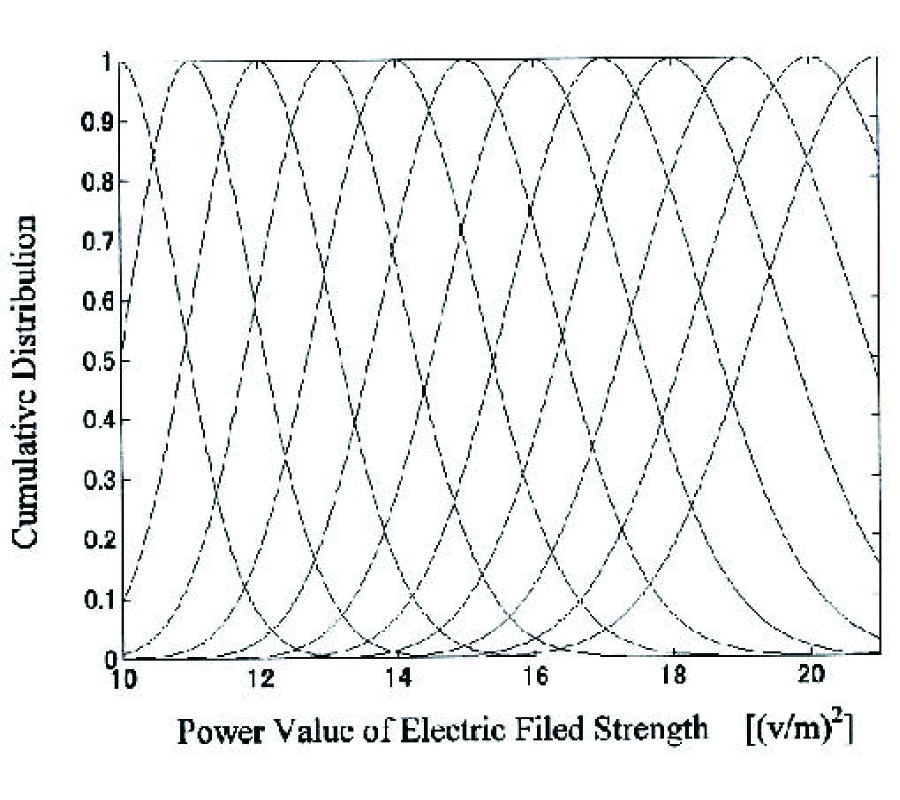2009-11-18
PIER B
Vol. 18, 347-363, 2009
Robust Semi-Deterministic Facet Model for Fast Estimation on EM Scattering from Ocean-Like Surface
Hui Chen , Min Zhang , Ding Nie and Hong-Cheng Yin
A robust semi-deterministic facet model for the computation of the radar scattering cross section from the ocean-like surface is presented. As a facet-based theory, it is a more comprehensive model which can reflect the specular and diffuse configurations, as well as the mono- and bistatic features. Significant computational efficiency and good agreement with experimental data are observed, which makes the proposed facet model well suitable for fast estimation on EM scattering and synthetic aperture radar(SAR) imagery simulation of marine scene.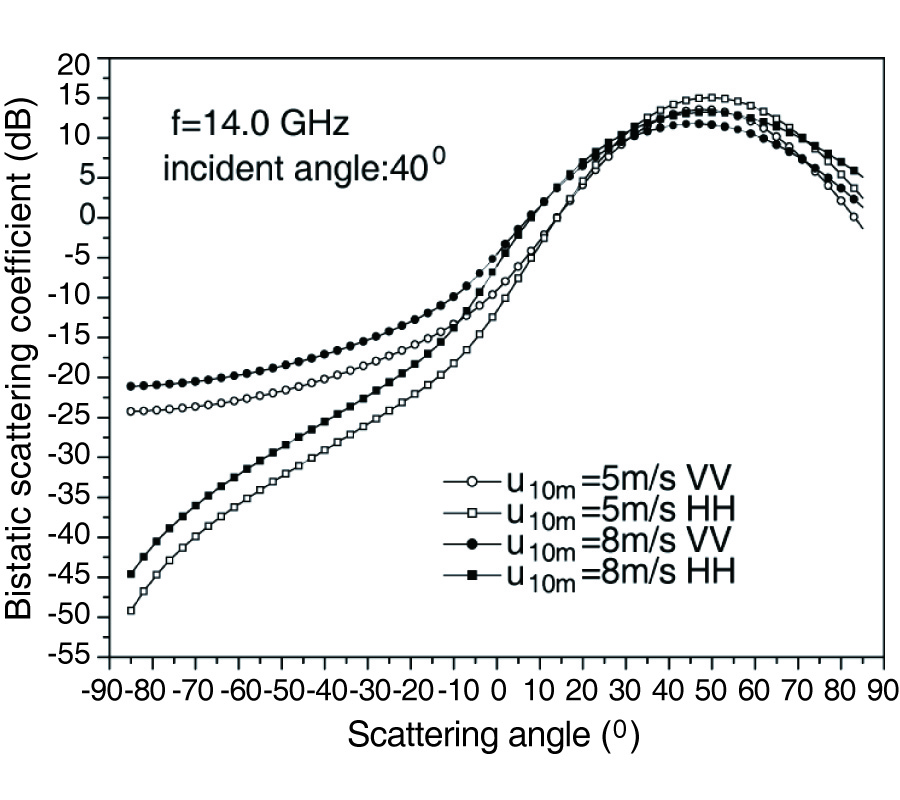2009-11-08
PIER B
Vol. 18, 327-346, 2009
Design of a Beam Forming Dielectric Cylindrical EBG Antenna
Claudio Biancotto and Paul Record
A novel dielectric cylindrical electronic bandgap antenna is presented and analysed using an in-house developed Finite-Difference-Time-Domain software simulator. The design steps and the simulations results of a geometrical parametric study are also presented and discussed, focusing on the design of antennas to operate in the X-band with high directivity patterns on the H-plane. Finally, the measurements results of a set of experiments carried out on a prototype showed very good agreement with simulations: 11% fractional bandwidth at 10 GHz and an average gain of 9.5 dBi are achieved in the impedance bandwidth with 13 dB front-to-back-ratio in the azimuthal plane.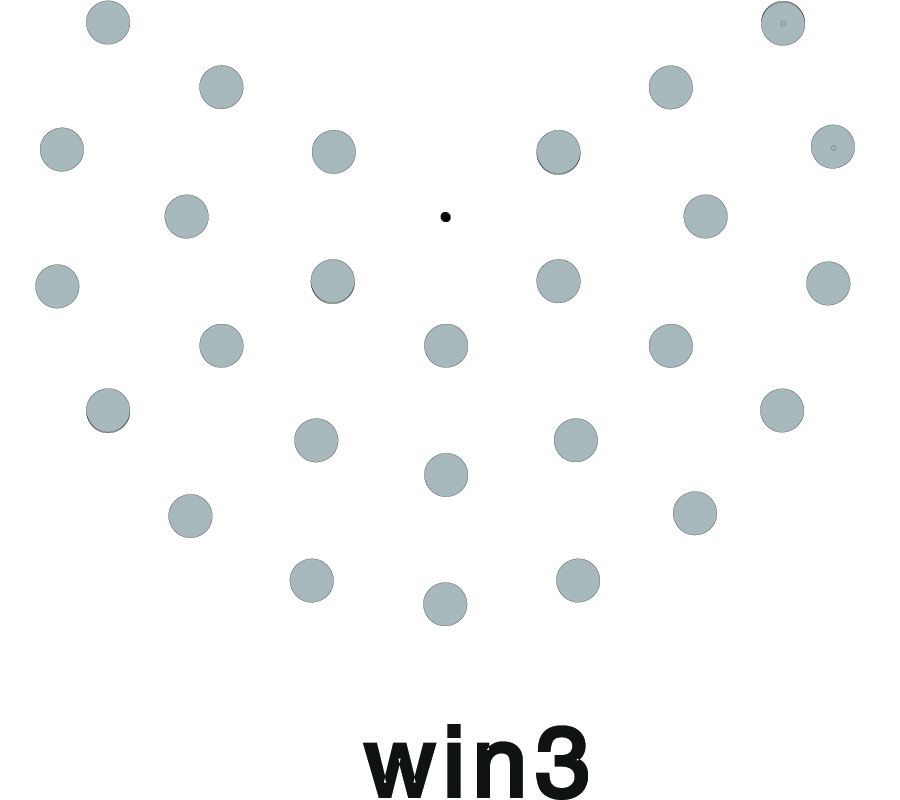2009-11-03
PIER B
Vol. 18, 311-325, 2009
Photonic Bandgaps in Quasiperiodic Multilayer Structures Using Fourier Transform of the Refractive Index Profile
Saeed Golmohammadi , Y. Rouhani , Karim Abbasian and Ali Rostami
In this paper, photonic bandgaps (PBGs) of the quasiperiodic structures is calculated using the Fourier transform of the refractive index profile. Comparing the reflectivity and Fourier spectrum of multilayer structure refractive index, we find that a peak in the Fourier spectrum is equivalent to a sinusoidal term in the refractive index. The wavelength of the peak location in the Fourier spectrum is half the wavelength where a PBG is located. Using Fourier transform analysis of the refractive index of any multilayer structure, we can determine the location of the PBGs of that structure. Peaks in the Fourier spectrum can be used to design reflective band optical filters in optical communication systems. The filtering wavelengths are twice the peaks in the Fourier spectrum.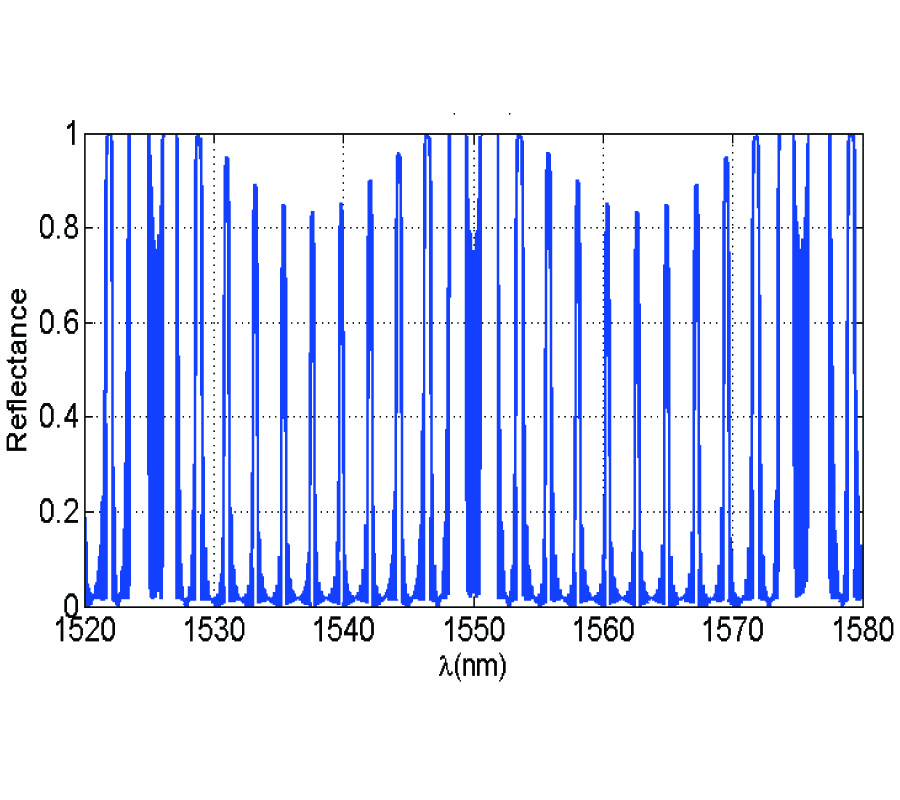2009-10-27
PIER B
Vol. 18, 279-309, 2009
Accelerated Antenna Design Methodology Exploiting Parameterized Cauchy Models
George Shaker , Mohamed H. Bakr , Nagula Sangary and Safieddin Safavi-Naeini
We propose an optimization methodology suitable for the design of various antenna structures. This methodology includes a rapidly-converging iterative scheme. In each iteration stage, the algorithm generates a parameterized Cauchy model using the available results from previous iterations. Optimization is then applied to this Cauchy model to obtain better design parameters that are also used in enhancing the accuracy of the model. This cycle continues until the specifications are met. In addition, this on-the-fly technique produces an analytical model of the behavior of the antenna structure. Sensitivity and tolerance analyses can thus be efficiently carried out without the need for further costly electromagnetic simulations.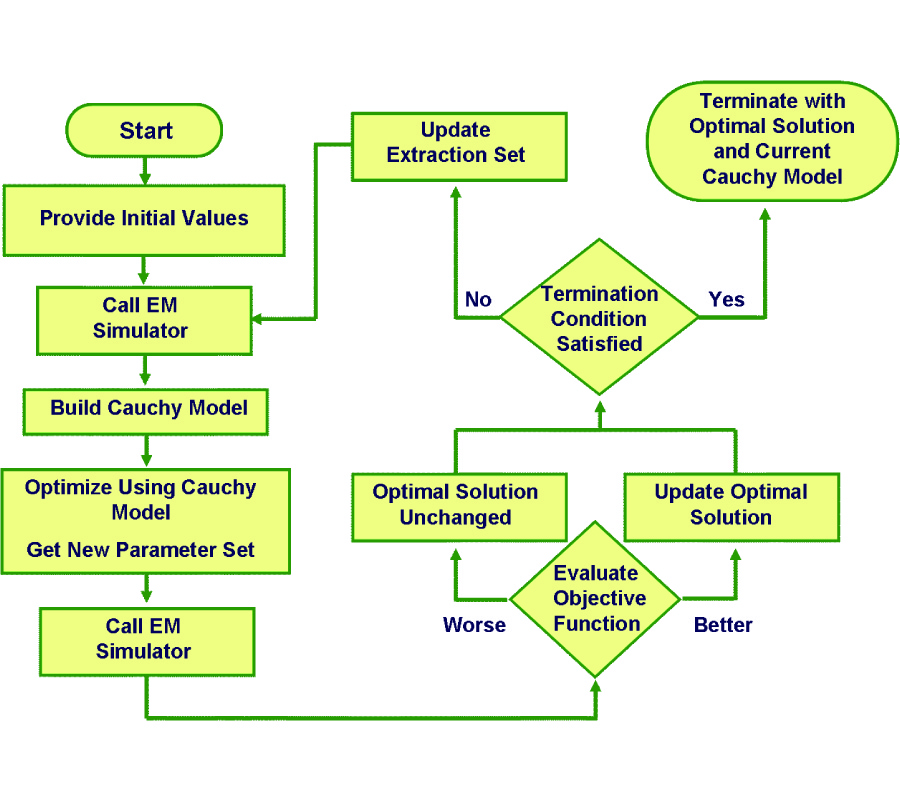2009-10-17
PIER B
Vol. 18, 259-277, 2009
Combined Strategies Based on Matrix Pencil Method and Tabu Search Algorithm to Minimize Elements of Non-Uniform Antenna Array
Shiyou Yang , Yanhui Liu and Qing Huo Liu
The minimization of elements in a non-uniform antenna array is critical in some practical engineering applications such as satellite and mobile communications. However, due to the complexity in the synthesis of an antenna array, the available techniques are not equally successful for reducing the element number of a non-uniform antenna array with as few elements as possible with respect to both solution quality and solution efficiency. In this point of view, a combined strategy based on the matrix pencil method and tabu search algorithm is proposed with the goal of integrating the advantages of the high solution efficiency of the matrix pencil method and the strong global searching ability of the tabu search algorithm when solving an antenna array design problem. In the proposed strategies, the desired radiation pattern is firstly sampled to form a discrete pattern data set. The matrix pencil method is then employed to optimize the excitations and location distributions of the antenna array elements to reduce the element number. Finally, the excitation and location distributions of antenna array elements are (repeatedly) re-optimized by using a tabu search algorithm by starting from the solution of the matrix pencil method to efficiently find the global solution of the design problem. To make the tabu search algorithm suitable for solving antenna array designs, some innovative approaches such as the elimination of the tabu list, systematic diversification as well as intensification processes for neighborhood creations are made. Numerical examples have shown the effectiveness and advantages of the proposed combined strategies.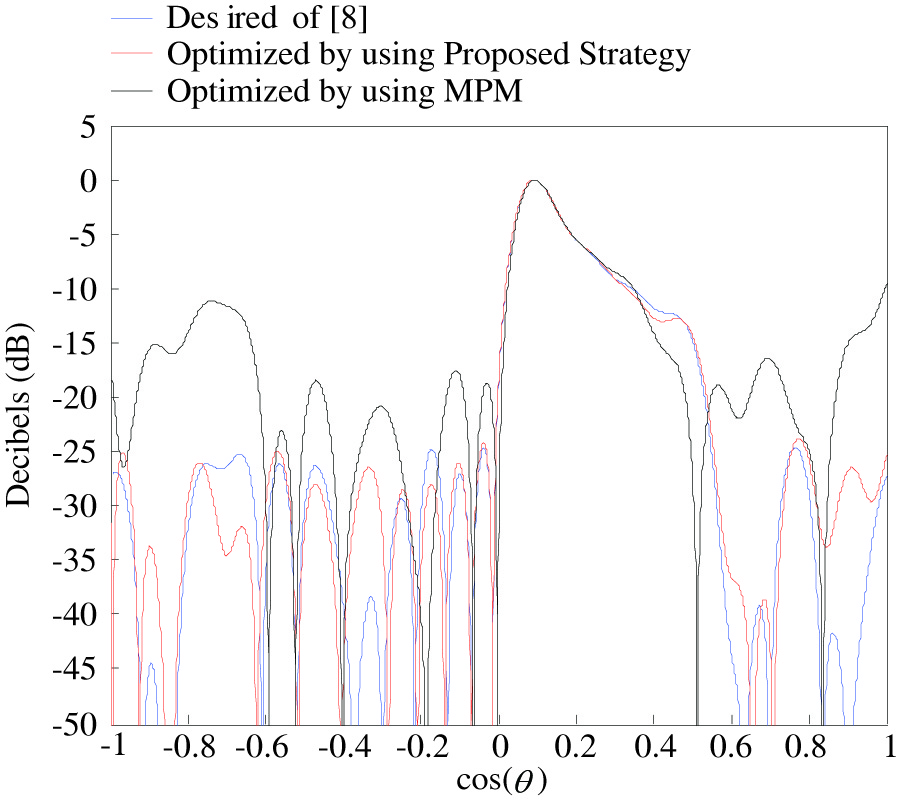2009-10-14
PIER B
Vol. 18, 243-257, 2009
A General FDTD Algorithm Handling Thin Dispersive Layer
Bing Wei , Shi-Quan Zhang , Yu-hang Dong and Fei Wang
A novel general technique for treating electrically thin dispersive layer with the finite difference time domain (FDTD) method is introduced. The proposed model is based on the modifying of the node update equations to account for the layer, where the electric and magnetic flux densities are locally averaged in the FDTD grid. Then, based on the characteristics that the complex permittivity and permeability of three kinds of general dispersive media models, i.e., Debye model, Lorentz model, Drude model, the permittivity and permeability can be formulated by rational polynomial fraction in , the conversion equation from frequency domain to time domain (i.e., replaced by ∂/∂t) and the shift operator method are then applied to obtain the constitutive relation at modified electrical points and the time-domain recursive formula for D and E, B and H available for FDTD computation are obtained. Several numerical examples are presented, indicating that this scheme possesses advantages such as fine generalization, EMS memory and time step saving and good precision.2009-10-12
PIER B
Vol. 18, 225-241, 2009
Scattering from Three-Dimensional Dispersive Gyrotropic Bodies Using the TLM Method
Ahmet Fazil Yagli , Jay Kyoon Lee and Ercument Arvas
A three-dimensional scattering field Transmission Line Modeling (TLM) algorithm is established to obtain bistatic radar cross sections of frequency dispersive gyromagnetic objects. Starting from the 1D TLM modeling of gyrotropic materials, a scattering field TLM algorithm is derived for 3D calculations. For verification, the bistatic radar cross section results for several gyromagnetic structures are compared with the single frequency computations, where the permittivity and permeability tensors are made of complex constants at a given frequency.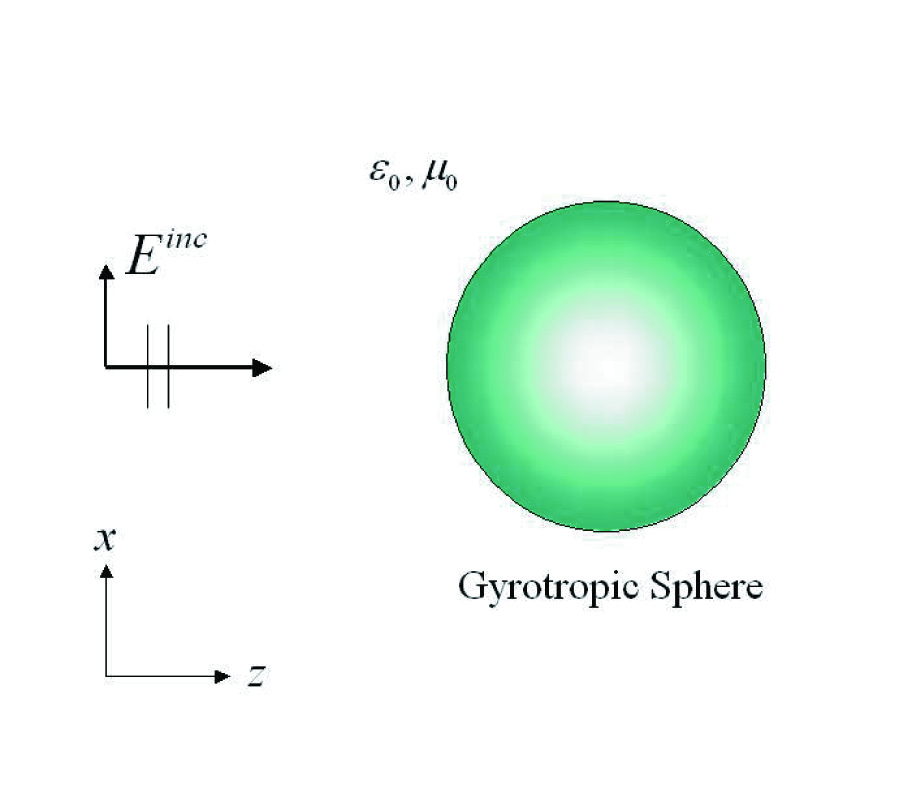2009-10-09
PIER B
Vol. 18, 205-224, 2009
Resonance on Transformers Excited by Square Waves and Explanation of the High Voltage on Tesla Transformer
Eduard Montgomery Meira Costa
This paper presents an analysis about resonance on coupled systems when excited by square waves, generated through experiments using planar and ring coils. Because of the phenomenon described by its transfer function, a sum of responses appear when the square wave frequency increases, which causes a resonance response with high voltage, in several cases, greater than common turn ratio of the transformer. With parallel capacitances inserted on output, the resonance frequency reduction and change of gain is observed. Due to this effect, explanation of how output of Tesla transformer presents high voltage which is shown, and strategies to reach the maximal value on output are proposed.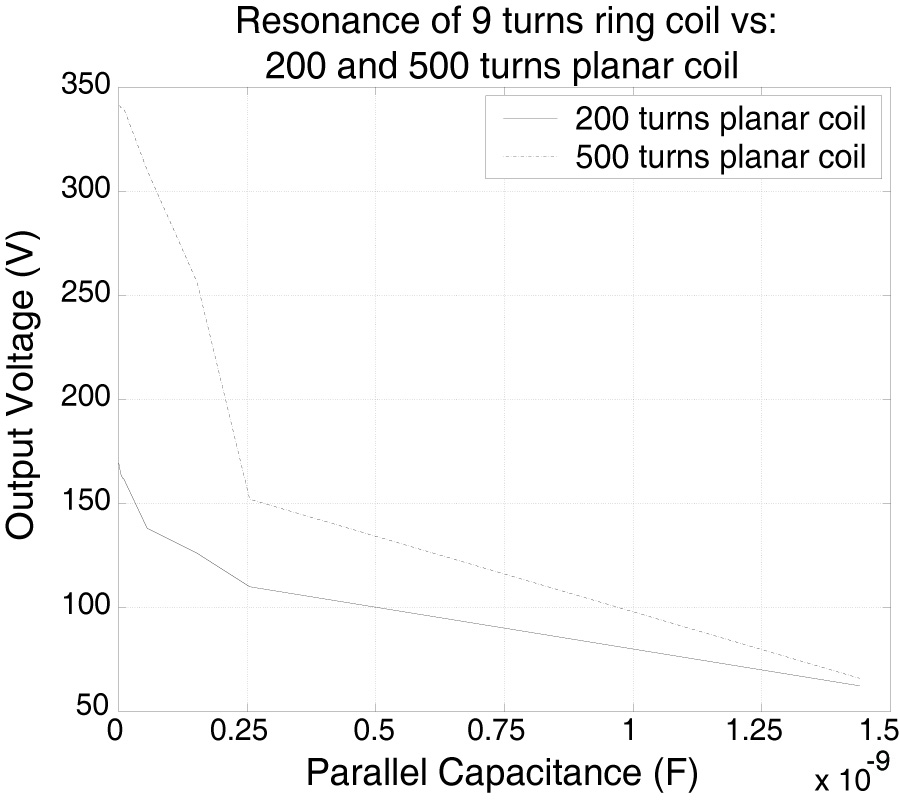2009-10-07
PIER B
Vol. 18, 185-204, 2009
Fet Small Signal Modelling Based on the Dst and Mel Frequency Cepstral Coefficients
Rania R. Elsharkawy , El-Sayed M. El-Rabaie , M. Hindy , Rreda Salama Mohamed Ghoname and Moawad Ibrahim Dessouky
In this paper, a new technique is proposed for field effect transistor (FET) small-signal modelling using neural networks. This technique is based on the combination of the Mel frequency cepstral coefficients (MFCCs) and discrete sine transform (DST) of the inputs to a neural network. The input data sets to traditional neural systems for FET small-signal modelling are the scattering parameters and corresponding frequencies in a certain band, and the outputs are the circuit elements. In the proposed approach, these data are considered as forming random signals. The MFCCs of the random signals are used to generate a small number of features characterizing the signals. In addition, other MFCCs vectors are calculated from the DST of the random signals and appended to the MFCCs vectors calculated from the signals. The new feature vectors are used to train the neural networks. The objective of using these new vectors is to characterize the random input sequences with much more features to be robust against measurement errors. There are two benefits for this approach: a reduction in the number of neural networks inputs and hence a faster convergence of the neural training algorithm and robustness against measurement errors in the testing phase. Experimental results show that the proposed technique is less sensitive to measurement errors than using the actual measured scattering parameters.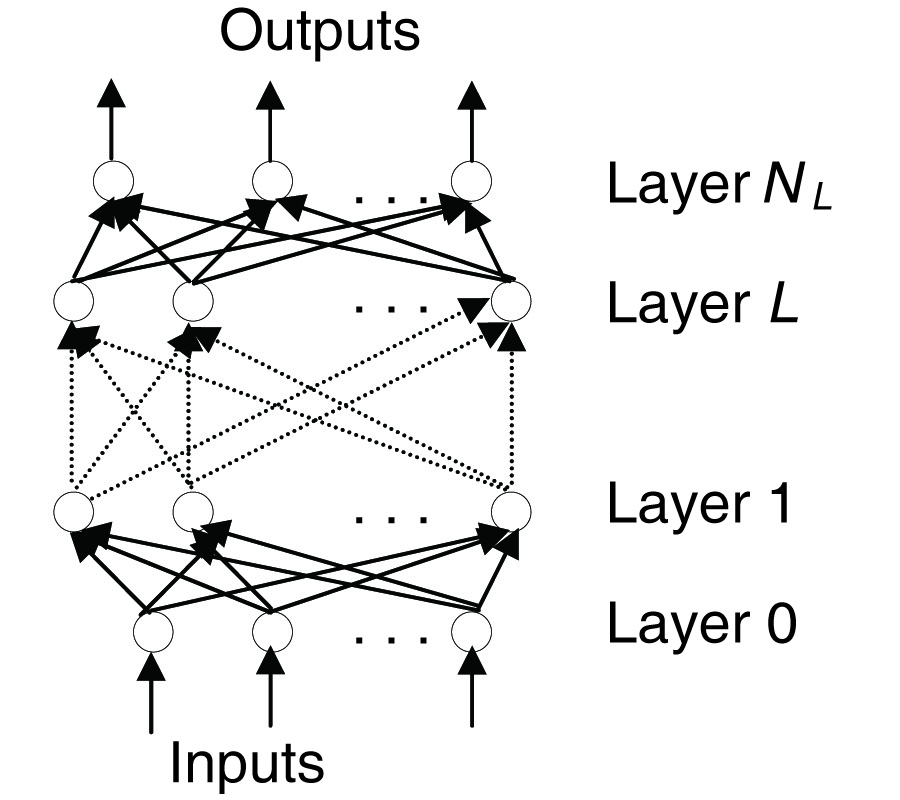2009-10-05
PIER B
Vol. 18, 165-183, 2009
Electromagnetic Source Transformations and Scalarization in Stratified Gyrotropic Media
Patrick De Visschere
It is known that with restrictions on the type of the constitutive equations, Maxwell's equations in non-uniform media can sometimes be reduced to two 2nd order differential equations for 2 scalar quantities only. These results have previously been obtained in two quite different ways, either by a "scalarization of the sources", where the relevant scalar quantities are essentially vector potential components, and the derivation was limited to isotropic media, or alternatively by using the "scalar Hertz potentials", and this method has been applied to more general media. In this paper it is shown that both methods are equivalent for gyrotropic media. We show that the scalarization can be obtained by a combination of transformations between electric and magnetic sources and gauge transformations. It is shown that the method based on the vector potential, which previously used a non-traditional definition of the vector potentials, can also be obtained using the traditional definition provided a proper gauge condition is applied and this method is then extended from isotropic to gyrotropic media. It is shown that the 2 basic scalar Hertz potentials occurring in the second method are invariant under the source scalarization transformations of the first method and therefore are the natural potentials for obtaining scalarization. Finally it is shown that both methods are also equivalent with a much older third method based on Hertz vectors.
2009-10-03
PIER B
Vol. 18, 149-163, 2009
Transparent Shells --- Invisible to Electromagnetic Waves
Zhong-Lei Mei and Tie-Jun Cui
In this paper, we study metamaterial-based transparent shells, which are invisible to electromagnetic waves and fields. More general topics, including material loss, material discretization and far field distribution etc. are covered in order to facilitate possible realization. We design and analyze the transparent shells using optical transformation. Unlike the widely-studied cloaking devices which make the objects inside invisible, the transparent shells physically cover and shield the devices inside without sacrifice of their electrical performance since they are transparent to incoming electromagnetic waves. Due to the simple constitutive parameters, these transparent structures could be realized using artificial metamaterials in a wide frequency band, which may have wide applications in civil and military areas.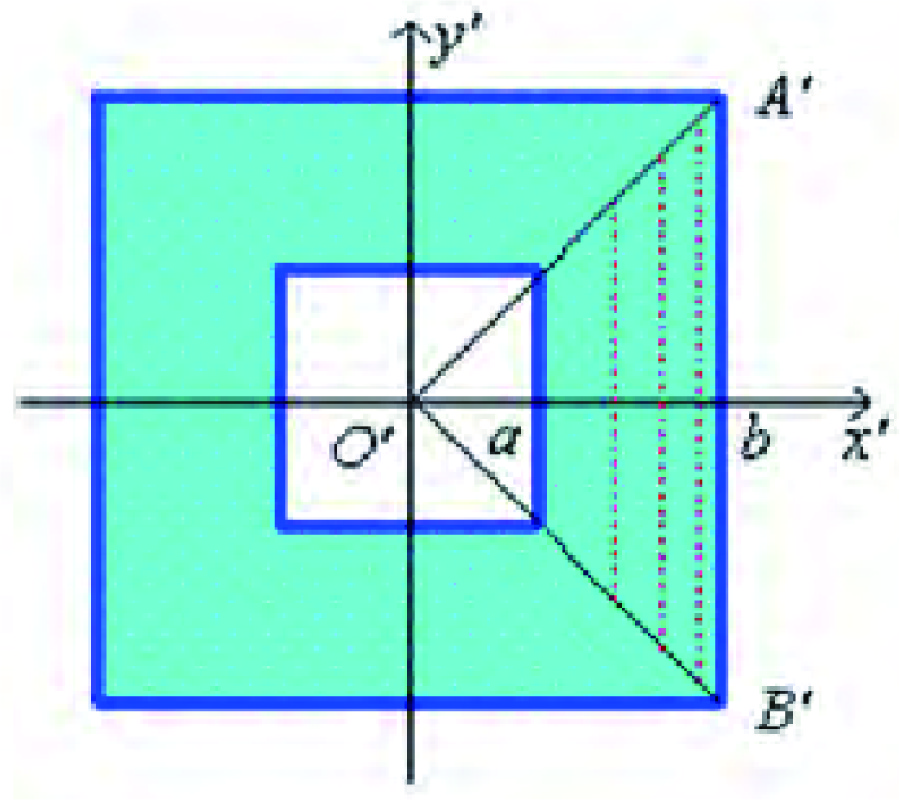2009-09-30
PIER B
Vol. 18, 121-148, 2009
Performance Evaluation of the Sequential Track Initiation Schemes with 3D Position and Doppler Velocity Measurements
Faruk Kural , Feza Arikan , Orhan Arikan and Murat Efe
This study investigates the effect of incorporating Doppler velocity measurements into the most commonly used sequential track initiation schemes, the rule and logic based schemes. The measurement set has been expanded from range and azimuth to include elevation and Doppler velocity. Unlike previous studies, elevation and Doppler measurements have also been included in the analytical evaluation of the false track initiation probability. Performance improvement obtained by employing Doppler measurements has been demonstrated through simulations in terms of false track initiation probability and true track initiation probability. Receiver Operating Characteristics and System Operating Characteristics have also been utilized in performance evaluation. Analytical derivations and simulations have revealed that, using Doppler velocity measurements along with 3D position measurements while initiating tracks in clutter leads to significant decrease in false track initiation probability while providing an acceptable level of true track initiation probability. Simulation results have also showed that inclusion of Doppler measurements has reduced the required time for track initiation, resulting in faster track initiation.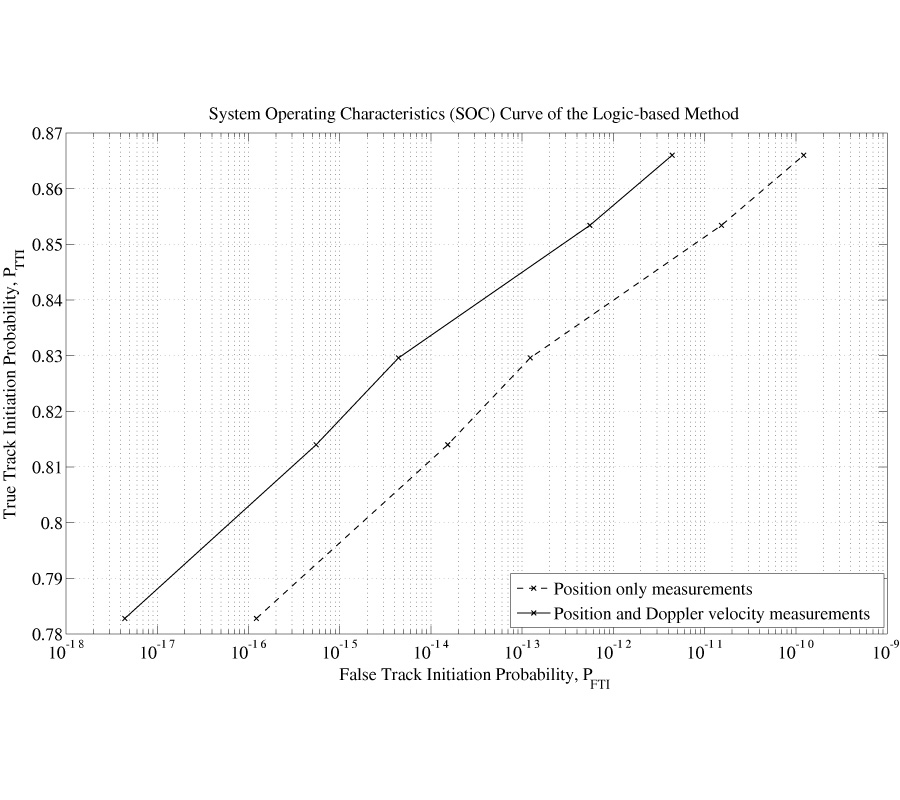2009-09-26
PIER B
Vol. 18, 101-119, 2009
Blind Joint DOA and DOD Estimation and Identifiability Results for MIMO Radar with Different Transmit / Receive Array Manifolds
Xiaofei Zhang , Xin Gao , Gaopeng Feng and Dazhuan Xu
This paper derives its link of direction of arrival (DOA) and direction of departure (DOD) estimation problem for multi-input multi-output (MIMO) radar to the trilinear model. With the exploitation of this link, we present a trilinear decomposition-based blind algorithm for angle estimation in MIMO radar. After the algorithmic presentation and discussions for identifiability results, trilinear decomposition-based angle estimation under different array conditions has also been investigated. Our proposed algorithm works well without either spectral peak searching or pair matching, has better angle estimation performance than ESPRIT, and even supports small sampling sizes. Simulation trials testify that the merits of our algorithmic performance are in collaboration with theoretical findings.2009-09-25
PIER B
Vol. 18, 83-99, 2009
Direction of Arrival Estimation Based on Fourth-Order Cumulant Using Propagator Method
Palanisamy Ponnusamy and Nageswara Rao
In this paper direction-of-arrival estimation (DOA) of multiple narrow-band sources, based on higher-order statistics using propagator, is presented. This technique uses fourth-order cumulants of the received array data instead of second-order statistics (auto-covariance) and then the so-called propagator approach is used to estimate the DOA of the sources. The propagator is a linear operator which only depends on the array steering vectors and which can be easily extracted from the received array data. But it does not require any eigendecomposition of the cumulant matrix of the received data like MUSIC algorithm. Computer simulations are carried out to compare the performance of the proposed method to those of methods based on auto-covariance using MUSIC and propagator algorithms.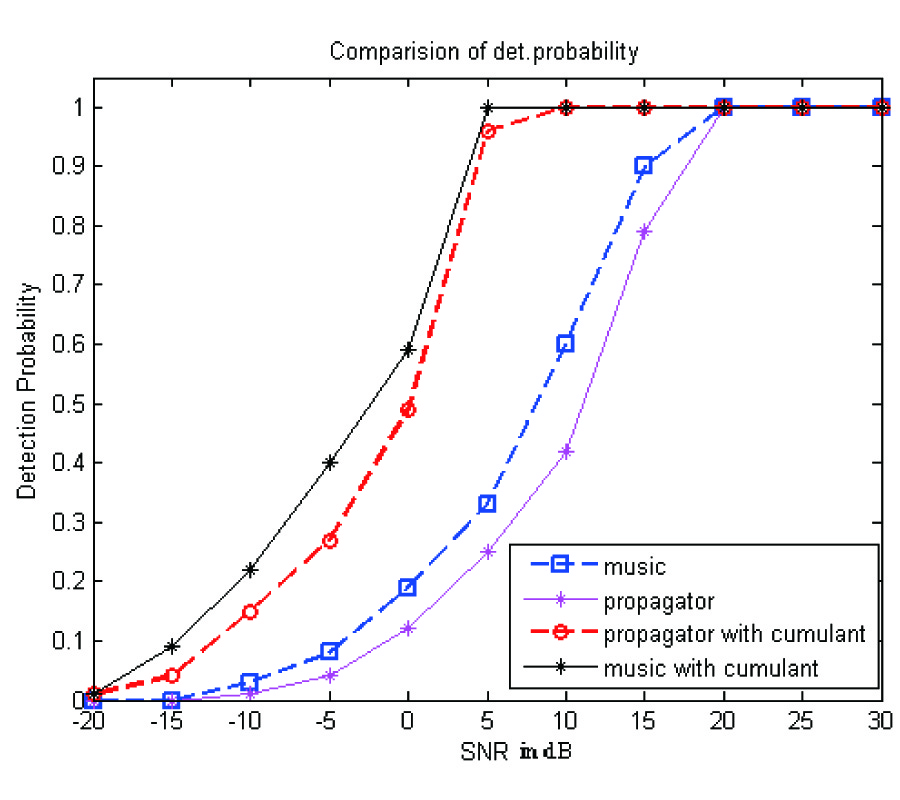2009-09-24
PIER B
Vol. 18, 59-81, 2009
Resonance Between Planar Coils Vs Ring Coils Excited by Square Waves
Eduard Montgomery Meira Costa
This paper presents a basic analysis about experiment results using planar coils inner ring coils, defining a transformer, when this system is excited by square wave voltage. In this study phenomena of response to step voltage due to parasitic capacitances is analyzed, and a sum of responses when the input square wave frequency increases is given too. These phenomena are uncommon in literature, which can be applied to several areas of researches in electrical engineering, pulse transformers and power electronics.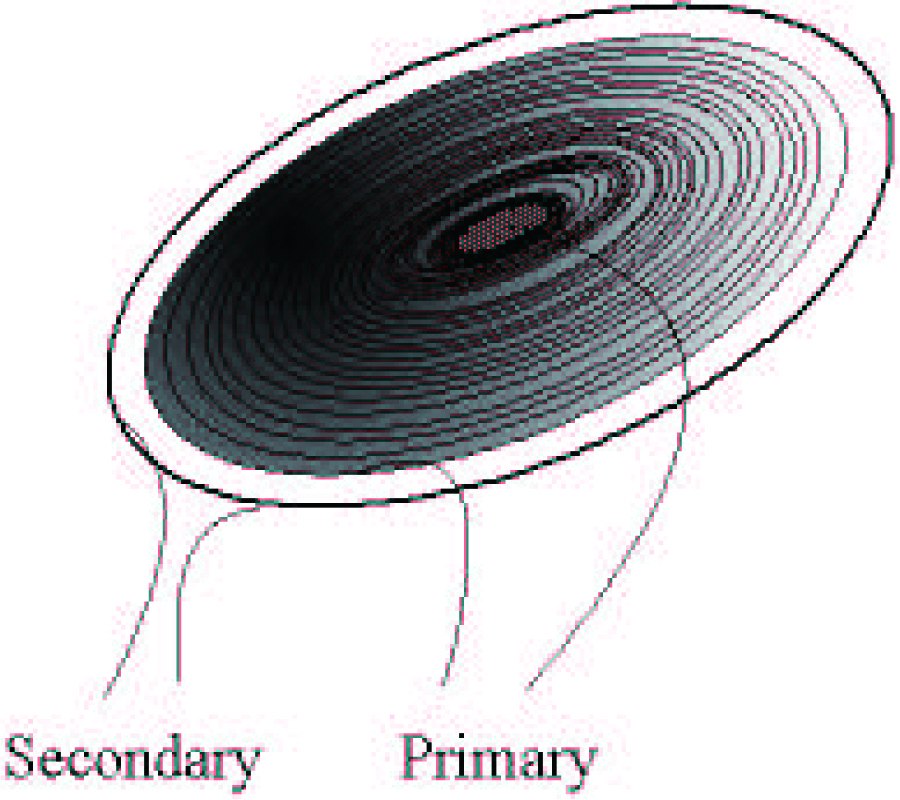2009-09-22
PIER B
Vol. 18, 43-58, 2009
Responses in Trasnformers Built with Planar Coils Inner Ring Coils Excited by Square Waves
Eduard Montgomery Meira Costa
This paper presents an analysis about the results of experiments using planar coils inner ring coils to determine a transformer. The excitation of this system was a square wave voltage, where experiments were realized in two ways: considering planar coil as primary and observing responses on secondary being ring coil (direct system) and the inverse (ring coil as primary and planar coil as secondary-inverted system). In this study, a phenomenon not common in literature on system response is analyzed, showing effects of changes on transfer function in both cases when varying turn numbers of each coil and several variations on responses due to changes on resistances, parasitic capacitances, self and mutual inductances of the coils. The uncommon phenomenon appears as modulated response on specific turn ratio. The obtained results can be used to researches in areas as power electronics and pulse transformers.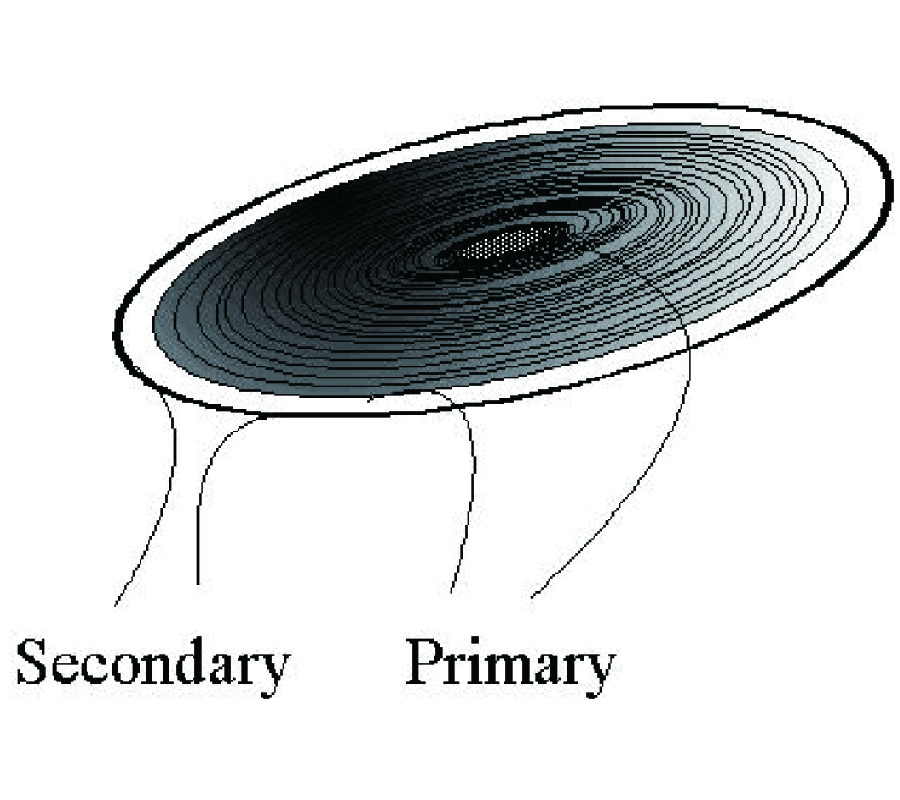2009-09-21
PIER B
Vol. 18, 25-42, 2009